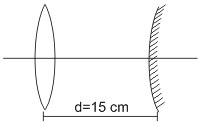# In an experiment a convex lens of focal length 15 cm is placed coaxially on an optical bench in front of a convex mirror at a distance of 5 cm from it. It is found that an object and its image coincide, if the object is placed at a distance of 20 cm from the lens. The focal length of the convex mirror is .Given focal length of lents (f) = 1.5 cm

Object distance u = - 20 cm ,  f = 15 cm

We know that

v = 60 cm

The image 1 gets formed at 60 cm to ghe right of the lens and it will be inverted.

Therefore, Distance between lens and mirror will be

d = image distance(v) - radius of curvature of convex mirror

s = 60 - 2f

2f = 60 - 5

(convex mirror)

Exams
Articles
Questions